# Riesz representation theorem

## Theorem

Let f be a bounded linear functional in a Hilbert space X over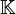$\mathbb K$. Then there exists a unique$y\in X$ such that, for all$x\in X$,

•$f(x)=\langle x,y\rangle$

and

•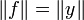$\lVert f\rVert =\lVert y\rVert$

## Proof

If f = 0 take y = 0. Suppose then that$f\neq 0$ and consider kerf, which is a closed space by this proposition. By the Hilbert space decomposition theorem we then have

•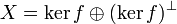$X=\ker f\oplus (\ker f)^\perp$

and, since$\ker f \not =X$,$(\ker f)^\perp$ has an element z such that$\lVert z\rVert =1$. Note now that, for all$x\in X$

•$f(x)z-f(z)x\in \ker f$

and, since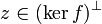$z\in (\ker f)^\perp$,

•$0=\langle f(x)z-f(z)x,z\rangle =f(x)-\langle x,\overline{f(z)}z\rangle$

that is to say

•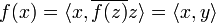$f(x)=\langle x,\overline{f(z)}z\rangle =\langle x,y\rangle$

with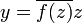$y=\overline{f(z)}z$. To prove uniqueness, suppose that for all$x\in X$

•$f(x)=\langle x,y_1\rangle =\langle x,y_2\rangle$

for some two elements$y_1,y_2\in X$. Then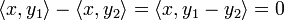$\langle x,y_1\rangle -\langle x,y_2\rangle=\langle x,y_1-y_2\rangle=0$ for all$x\in X$. In particular, for x = y1y2 which would imply$\lVert y_1-y_2\rVert ^2=0$ and hence y1 = y2. Finally, since the inner product is continuous on the first variable

•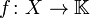$f\colon X\to \mathbb K$,$f(x)=\langle x,y\rangle$

is a linear functional that is continuous, and hence bounded by the bounded operator equivalence theorem. Applying the Cauchy-Schwarz inequality, we get

•$|f(x)| =|\langle x,y\rangle|\leq\lVert x\rVert\cdot\lVert y\rVert$

and so$\lVert f\rVert =\sup_{\lVert x\rVert =1}\; |f(x)|\leq \lVert y\rVert$. If y = 0 then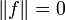$\lVert f\rVert=0$ so f = 0. If not, consider$z=\lVert y\rVert^{-1}y$ to obtain

•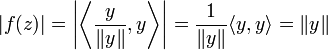$|f(z)|=\left|\left\langle\frac{y}{\lVert y\rVert},y\right\rangle\right|=\frac{1}{\lVert y\rVert}\langle y,y\rangle=\lVert y\rVert$

which yields$\lVert f\rVert=\lVert y\rVert$.Some content on this page may previously have appeared on Bourbawiki.